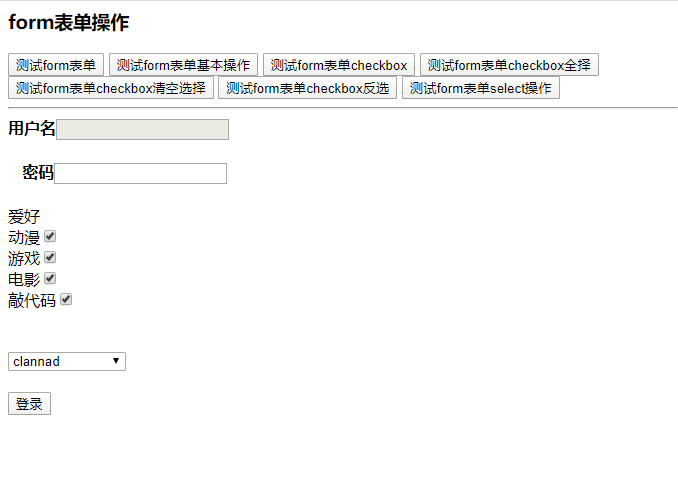Complete example of JS document form form element operation

Time：2020-7-13

This paper describes the operation of JS document form form elements. For your reference, the details are as follows:

<html>
< title > formform operation
<meta charset="UTF-8"/>
<script type="text/javascript">
function testForm(){
//The first way to get the form object is through ID, which is a common method
var fm=document.getElementById("fm");
Alert (FM); // output the type of FM [object htmlformelement]
alert( fm.length ）; // output the form item used (form type reference Lgin.html ）The output value is 8
//Method 2 of getting form object is a special method through name
var frm=document.frm;
Alert (FM = = = FRM); // the return value is true, which is the same in both type and content. It is the same object
}
function testOper(){
var fm=document.getElementById("fm");
//Output the elements of the form
//                alert( fm.elements ）; // displays the type of the form element [object htmlformcontrolscollection]
//                alert( fm.elements.length ）; // displays the number of form elements
//Dynamic change of access address by using action attribute of form
fm.action="http://www.baidu.com";
alert( fm.action ）; // returns the value of action
//Using the submit attribute of the form to make the normal button also have the submission function
//                 fm.submit (); // you can visit the modified website directly
//                alert( fm.submit ()); // submitted and undefined returned
}
function testCheck(){
//Get the object of the check box
var fav= document.getElementsByName ("fav"); // cannot be obtained by ID, but by name
//Implementation of selected traversal print operation
for(var i=0;i<fav.length;i++){
if(fav[i].checked){     //
Alert (fav [i]. Value); // where fav has no value, but fav.value With value, fav cannot be printed, but can be printed fav.value
}
}
}
//Realize the operation of all selection
function checkBoxselect(){
var fav=document.getElementsByName("fav");
for(var i=0;i<fav.length;i++){
fav[i].checked=true;
}
}
//Realize the empty selection operation
function checkBoxclear(){
var fav=document.getElementsByName("fav");
for(var i=0;i<fav.length;i++){
fav[i].checked=false;
}
}
//Realize the reverse selection operation
function checkBoxchange(){
var fav=document.getElementsByName("fav");
for(var i=0;i< fav.length ; I + +) {/ = is the evaluator = = and = = = are the judges
if(fav[i].checked){
fav[i].checked=false;
}else{
fav[i].checked=true;
}
}
}
function selectoper(){
var sel=document.getElementById("Animation");
//Gets the options in the radio box
var os=sel.options;
for(var i=0;i<os.length;i++){
if(os[i].selected){
}
}
}
</script>
<body>
<h3>Form form operation</h3>
<! -- adding readonly means that the tag can only be read, and adding the disabled tag means that the tag can not be operated on -- >
< input type = "button" name = value = test form "onclick =" testform() "/ >
< input type = "button" name = value = test form basic operation "onclick =" testoper() "/ >
< input type = button "name = value = test form check box" onclick = "testcheck()" / >
"," check type = "check box()" to test
< input type = "button" name = "value =" test form checkbox empty selection "onclick =" checkboxclear() "/ >
< input type = "button" name = "value =" test form checkbox reverse selection "onclick =" checkboxchange() "/ >
< input type = "button" name = value = test form select operation "onclick =" selector() "/ >
<hr />
<form action="#" method="get" name="frm" target="_blank">
<b>User name < / b > < input type = "text" name = "WD" value = "disabled" / > < br / > < br / > < br / >
<b>Password < / b > < input type = "password" name = "PWD" value = "readonly =" readonly "/ > < br / >
<br />
Hobbies < br / >
Animation < input type = "check box" name = "fav" value = "animation" checked = "false" / > < br / >
Game < input type = "check box" name = "fav" value = "game" checked = "false" / > < br / >
Movie < input type = check box "name =" fav "value = movie" checked = true / > < br / >
Typing Code < input type = checkbox "name =" fav "value = Typing Code" checked = "false" / > < br / >
<br /><br />
<select name="">
< option value = > flower story < / option >
< option value = > unknown flower name < / option >
< option value = > April is your lie < / option >
</select>
<br /><br />
< input type = "submit" name = value = "login" / >
</form>
</body>
</html>

Operation effect:Interested friends can useOnline HTML / CSS / JavaScript code running tool: http://tools.jb51.net/code/HtmlJsRun Test the above code running effect.

More about JavaScript related content can see our special topic: “JavaScript operation DOM skills summary”, “JavaScript page element operation skills summary”, “JavaScript event related operations and skills”, “JavaScript search algorithm skills summary”, “JavaScript data structure and algorithm skills summary”, “JavaScript traversal algorithm and skills summary” and “javasc” Ript errors and debugging skills summary